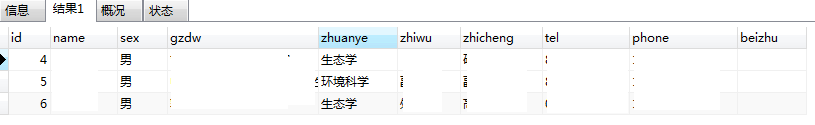MySQL如何实现随机查询时有对查询条件的判断?

## 首先,实现随机的方法我知道.问题是如何在查询随机数据时能够对条件进行判断

### 举个例子• 先查询出所有的数据(随机)
• 再通过一个存贮函数来判断条件是否达到
• 达到后,结束

通过下面的代码,能够查询出每个专业的人数,可以用来做判断,是否满足条件,但是这是查询全部的.

当我想要查询只要10个人时,下面的代码查的是所有人.

但是,我要是先随机查询出10个人,那么这10个人不一定会满足条件.如果一直随机查询直到满足条件,那就太浪费资源了

``````SELECT  COUNT(t.zhuanye) c
FROM t_expert AS t
GROUP BY t.zhuanye
HAVING c>=2
``````

4个回答

• 在数据量比较大的时候,不能将所有的数据都一次性取出来.只能取一部分.
• 而这一部分数据在遍历完之后,可以能也不会满足需求.
• 这时,还是需要再去取数据.又增加了数据库的访问次数.而且还不一定需要多少次.

2018-12-19``````SELECT * FROM test WHERE id >= (SELECT floor(RAND() * (SELECT MAX(id) FROM test)));
``````

``````SELECT * FROM test WHERE id >= (SELECT floor(RAND() * (SELECT MAX(id) FROM test))) ORDER BY id LIMIT 0,5;

``````Roobert_Chao 回复居十四: 在查询的时候约束条件设置满足后可以查询出一些内容，还可以对sql 中闯入一个数组，这个数组 中存随机数，然后再根据随机数查询。1.最简单的办法order by rand(),示例

select * from question q where q.`level`=1 order by rand() limit 1;

2.如果记录id保持连续增长，中间不间断，则可以用其它方式替代上述语句，示例

#随机查询（记录大于某个数，效率高）
select q1.* from question q1 inner join (select (min(q2.id) + round(rand()*(max(q2.id) - min(q2.id)))) as id from question q2 where q2.`level`=1) as t
on q1.id >= t.id limit 1;

#效率略低
select q.* from question q where q.id > (select t.id from (
select (min(q2.id) + round(rand()*(max(q2.id) - min(q2.id)))) as id from question q2 where q2.`level`=1
) t) limit 1;

#效率极低，比order by rand还低（可能针对每条记录都作了子查询，结果不不连续，很随机）
select q.* from question q where q.id > (select (min(q2.id) + round(rand()*(max(q2.id) - min(q2.id)))) from question q2 where q2.`level`=1) limit 1;

select q1.* from question q1 inner join (select (min(q2.id) + round(rand()*(max(q2.id)-2 - min(q2.id)))) as id from question q2 where q2.`level`=1) as t
on q1.id >= t.id limit 3;

select q1.* from question q1 inner join (
select (min(q2.id) + round(rand()* ( case when (max(q2.id)-2)>min(q2.id) then max(q2.id)-2 - min(q2.id) else 0 end ))) as id,min(q2.id) as minId,max(q2.id) as maxId from question q2 where q2.`level`=2
) as t
on q1.id >= t.id and q1.id between t.minId and t.maxId limit 3;

select 10*RAND(); #[0,10)
select FLOOR(10*RAND());#[0,9]
select CEILING(10*RAND()); #[1,10]
select ROUND(10*RAND()); #[0,10]